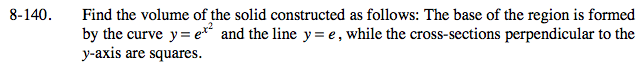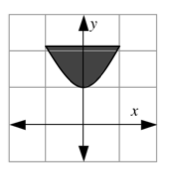Home > CALC > Chapter 8 > Lesson 8.4.1 > Problem8-140

8-140.Start by sketching the base of the solid. Notice the x- and y- coordinates where the functions intersect. Also notice the bottom-most point on the solid. Visualize the square cross-sections that will stand up upon this base, making a 3D figure. Each cross-section is perpendicular to the y axis, which means they will be horizontally aligned. At the bottom, near y = 1, the squares will be very small. As you move up, they will gradually get larger. The largest square, at y = e, will have a side length of 2.

The bounds: Since the squares are horizontal, the bounds will be y-values. What are the lowest and the highest y-value?The integrand:
Since the squares are horizontal, the integrand must be written in terms of y.
In other words, solve for x.
y = ex2
ln(y) = x2ln(e)

Careful! Observe that, because of symmetry across the y-axis, x represents just half of the side-length of each square.

The integral:
Put it all together.

$\text{Volume by Cross Sections }=\int_{y=a}^{y=b}(\text{Area})dy$